SEARCH HOMEMath Central Quandaries & QueriesQuestion from Cecelia, a parent: I HAVE A SEMI CIRCULAR TANK AGAINST A WALL MEASURING 6'10" ACROSS THE WIDEST PART  AND 3' FROM THE WALL TO THE FRONT OF THE TANK.  THE DEPTH IS 14".  PLEASE HELP ME TO CALCULATE THE VOLUME OF WATER IN THE TANK.Hi Cecelia.

That sounds like an big aquarium. Based on your measurements, I think this sketch shows the situation: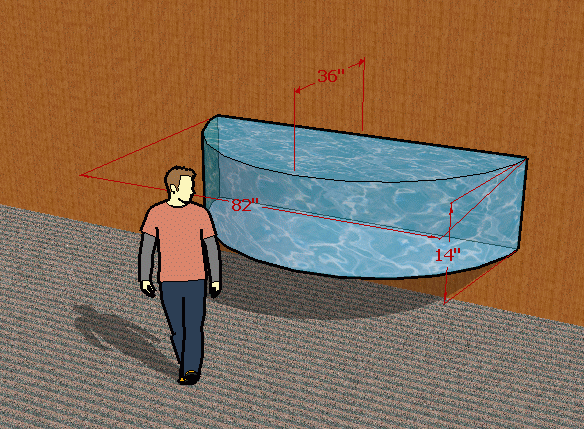Because the length along the wall is more than twice the distance from the wall to the front, you don't actually have a full semicircle, in which case the calculation would be easier. Instead, this is a chord of a circle with an unknown radius.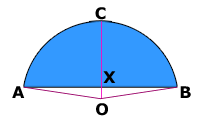Here is a top view.

The pink lines indicate radii from the three points on the tank to the implied center of the circle at point O. I've also labelled the other interesting spots we'll need to refer to.

The area in blue is a circular segment. The area of the circular segment is easiest to obtain by subtracting the area of the isoceles triangle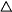OBA from the circular sector (pie slice) OBA. The volume of the tank is just the depth (14") times this blue area.

The area of a circular sector is ½r2θ where θ is the angle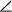AOB in radian measure and r is the length of any of the pink radii. The area of the isoceles triangle is ½(AB)(OX). But (OX) is just the radius r minus the length CX, which we know is 36 inches and AB is 6' 10" which is 82 inches. So the area ofOBA = ½(82)(r - 36). Thus the volume of the tank is V = (14) [ ½r2θ - ½(82)(r - 36) ] which reduces to

V = 7 [ r2θ - 82r + 36(82) ] .

So we need to find r and θ. Let's draw a more detailed diagram.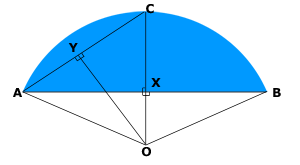Since OC bisectsAOB, and OY bisectsAOC, then we know thatYOC = ¼θ. Also, since CX bisects AB, we know the length AX = ½(82) = 41 inches.

This means we know the length of two sides ofAXC, so we can find AC by using Pythagorus:

AC2 = 412 + 362
AC = 54.58 inches.

Since OY bisects AC, then YC = ½(54.58) = 27.26 inches.

Also, since we know two sides ofAXC, we can find the measure ofXCA:XCA = tan-1 (41/36) = 0.850 radians.

And inOCY, we haveC, soO = (π/2) -C = 0.72055 radians, so θ = 4(0.72055) = 2.8822 radians.

As well, r = CO = YC / (cosXCA) = (27.26) / cos (0.850) = 41.3 inches.

So we can put these values of r and θ into our equation for the volume:

V = 7 [ (41.3)2(2.8822) - 82(41.3) + 36(82) ] = 30652 cubic inches.

I see you are writing from Jamaica and I am uncertain whether Jamaicans use liters, US gallons or UK gallons for measuring water volume. Respectively, their conversion factors give:

V = 502 liters or 132.6 US gallons or 110.4 UK gallons.

Stephen La Rocque.>Math Central is supported by the University of Regina and The Pacific Institute for the Mathematical Sciences.